GRE Subject Test: Math : Simpson's Rule

Example Questions

Example Question #1 : Simpson's Rule

Solve the integral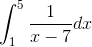using Simpson's rule with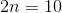subintervals.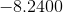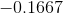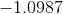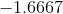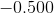Explanation:

Simpson's rule is solved using the formula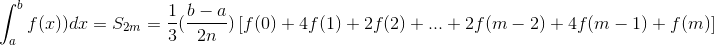whereis the number of subintervals and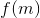is the function evaluated at the midpoint.

For this problem,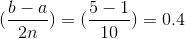.

The value of each approximation term is below.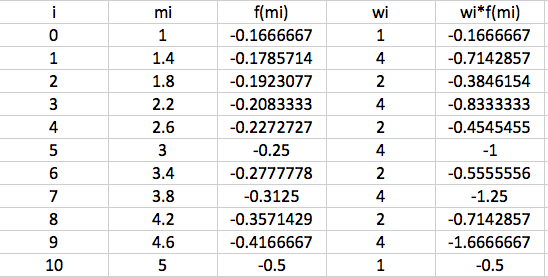The sum of all the approximation terms is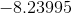therefore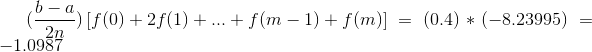Example Question #1 : Numerical Approximation

Solve the integral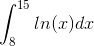using Simpson's rule withsubintervals.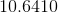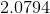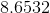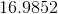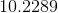Explanation:

Simpson's rule is solved using the formulawhere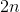is the number of subintervals andis the function evaluated at the midpoint.

For this problem,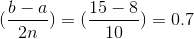.

The value of each approximation term is below.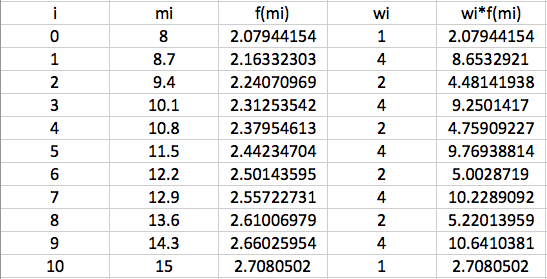The sum of all the approximation terms is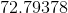therefore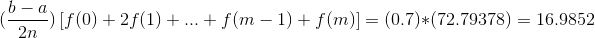Example Question #2 : Numerical Approximation

Solve the integral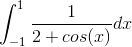using Simpson's rule withsubintervals.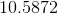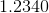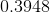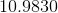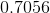Explanation:

Simpson's rule is solved using the formulawhereis the number of subintervals andis the function evaluated at the midpoint.

For this problem,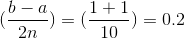.

The value of each approximation term is below.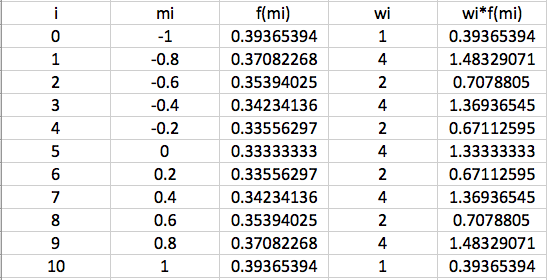The sum of all the approximation terms is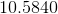therefore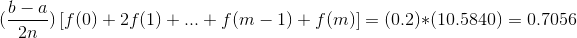Example Question #2 : Simpson's Rule

Solve the integral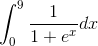using Simpson's rule withsubintervals.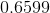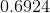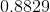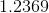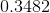Explanation:

Simpson's rule is solved using the formula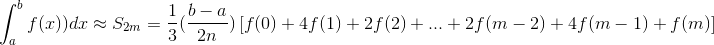whereis the number of subintervals andis the function evaluated at the midpoint.

For this problem,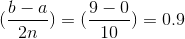.

The value of each approximation term is below.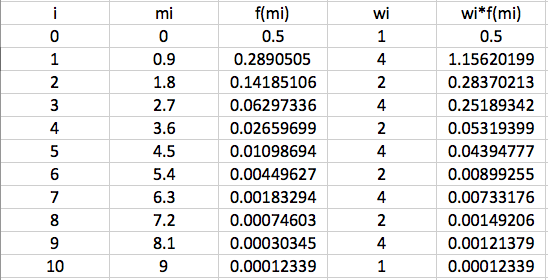The sum of all the approximation terms is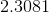therefore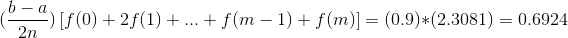All GRE Subject Test: Math Resources